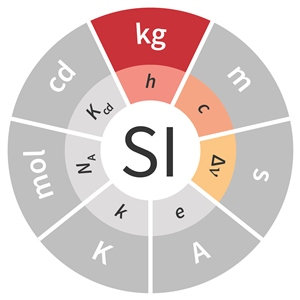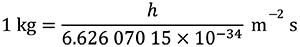# SI base unit: kilogram (kg)

The kilogram, symbol kg, is the SI unit of mass. It is defined by taking the fixed numerical value of the Planck constant h to be 6.626 070 15 x 10–34 when expressed in the unit J s, which is equal to kg m2 s–1, where the metre and the second are defined in terms of c and ΔνCs.This definition implies the exact relation h = 6.626 070 15 x 10–34 kg m2 s–1. Inverting this relation gives an exact expression for the kilogram in terms of the three defining constants h, ΔνCs and c:which is equal toThe effect of this definition is to define the unit kg m2 s–1 (the unit of both the physical quantities action and angular momentum). Together with the definitions of the second and the metre this leads to a definition of the unit of mass expressed in terms of the Planck constant h.